# A point dipole of dipole moment -p is located at the center of a grounded (Phi_Shell...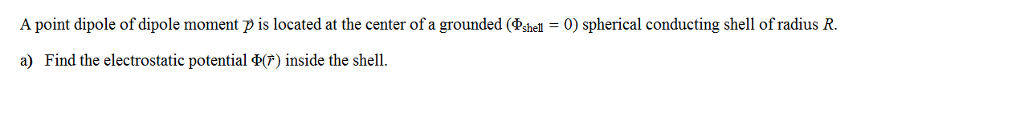A point dipole of dipole moment -p is located at the center of a grounded (Phi_Shell = 0) spherical conducting shell of radius R. a) Find the electrostatic potential Phi (F) inside the shell.

As the dipole moment p is placed at the center.

We know that, point dipole at origin will have the potential of

(1/4π).(p.cos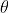/r2)

Laplace equation :

lrl+ Bl/rl+1

l= 1 is only relevant term ,( held at center)

V(r,)= (A1r1+ p/4π0r2)cos+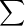Aerl-1plcos[ Ae=0]

V(r=R,)= A1R+ (p/4π)(1/R2)=0

V(r,)= p/4π0(1/r2-r/R3)cos#### Earn Coin

Coins can be redeemed for fabulous gifts.

Similar Homework Help Questions
• ### Potential Inside a grounded shell

A grounded &conducting spherical shellof outer radius M and inner radius N has a point charge q insidethe shell at a distance r<M from center. Using methodof images,find:a) the potential inside thesphere;b) the induced surface charge density on theinner surface of the shell at r=M; what is the total inducedcharge?c) the magnitude and direction of the forceacting on q. Does q get pushed towards the center, or away from thecenter?d) Is there any change in the solution if thesphere is...

• ### A point charge q is located a distance r from the center of a conducting shell...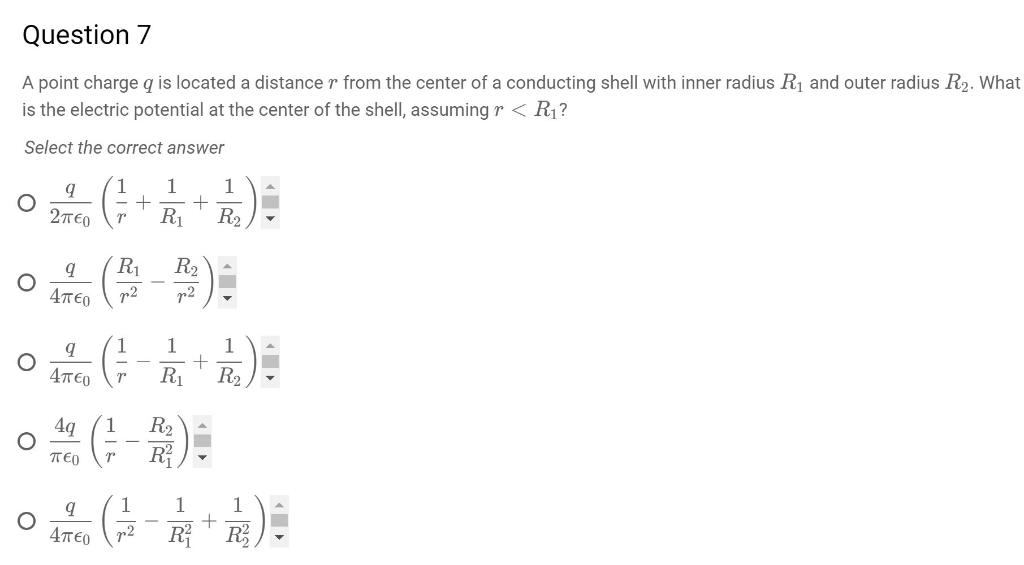A point charge q is located a distance r from the center of a conducting shell with inner radius R​1​​ and outer radius R​2​​. What is the electric potential at the center of the shell, assuming r <R​1​​? Question 7 A point charge q is located a distance r from the center of a conducting shell with inner radius R1 and outer radius R2. What is the electric potential at the center of the shell, assuming r< R1? Select the...

• ### A point charge is located at the center of a conducting spherical shell with inner radius...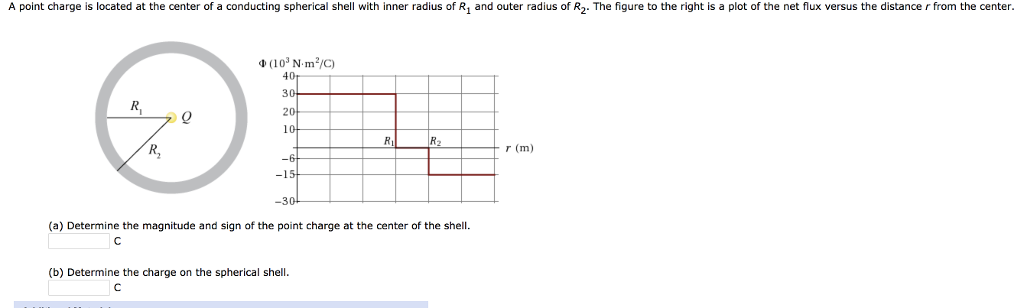A point charge is located at the center of a conducting spherical shell with inner radius of R1 and outer radius of R2. The figure to the right is a plot of the net flux versus the distance r from the center 40 30 10 R2 r (m) 30 (a) Determine the magnitude and sign of the point charge at the center of the shell. (b) Determine the charge on the spherical shell

• ### 3. Select the correct sketch of the direction of dipole moment of pair of charges a)...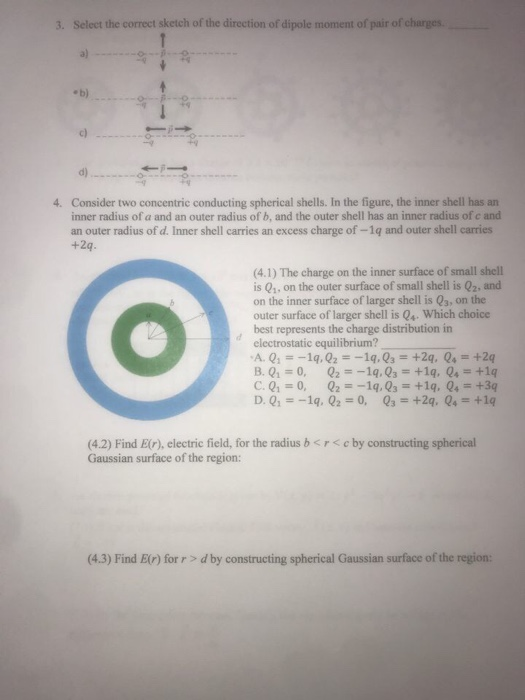3. Select the correct sketch of the direction of dipole moment of pair of charges a) e b) 19 c) d) Consider two concentric conducting spherical shells. In the figure, the inner shell has an inner radius of a and an outer radius of b, and the outer shell has an inner radius of c and an outer radius of d. Inner shell carries an excess charge of-1q and outer shell carries 4. (4.1) The charge on the inner surface...

• ### A positive point charge Q is located in free-space at the center of a spherical conducting...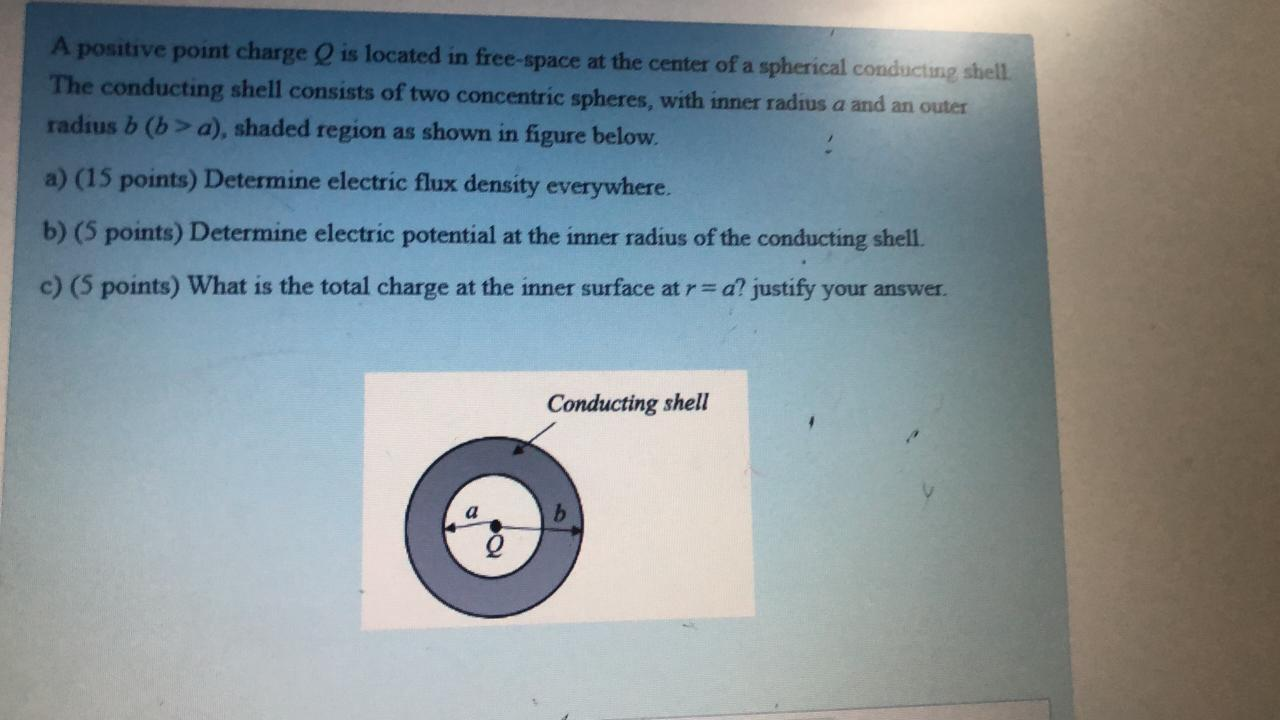A positive point charge Q is located in free-space at the center of a spherical conducting shell The conducting shell consists of two concentric spheres, with inner radius a and an outer radius b (b> a), shaded region as shown in figure below. a) (15 points) Determine electric flux density everywhere. b) (5 points) Determine electric potential at the inner radius of the conducting shell c) (5 points) What is the total charge at the inner surface at r=a? justify...

• ### A point charge q is located at the center of a spherical shell of radius a...

A point charge q is located at the center of a spherical shell of radius a that has a charge -q uniformly distributed on its surface. Find the electric field for the following points: (a) for all points outside the spherical shell E = kq/r2 E = kq2/r2 E = q/4pr2 none of these E = 0 (b) for a point inside the shell a distance r from the center E = q/4pr2 E = kq2/r2 E = kq/r2 E...

• ### A point charge q is located at the center of a spherical shell of radius a...

A point charge q is located at the center of a spherical shell of radius a that has a charge −q uniformly distributed on its surface. Find the electric field for the following points: (a) for all points outside the spherical shell E = q/4πr2 E = 0     E = kq2/r2 E = kq/r2 none of these (b) for a point inside the shell a distance r from the center E = kq2/r2 E = kq/r2     E = q/4πr2 none...

• ### Electrodynamics 5. Suppose an ideal electric dipole (what Griffiths calls a perfect dipole) of dipole moment...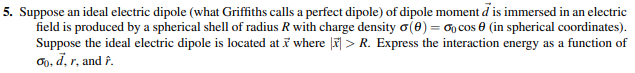Electrodynamics 5. Suppose an ideal electric dipole (what Griffiths calls a perfect dipole) of dipole moment d is immersed in an electric field is produced by a spherical shell of radius R with charge density (O) = Gocos (in spherical coordinates). Suppose the ideal electric dipole is located at x where > R. Express the interaction energy as a function of 00, d. r, and f.

• ### A dielectric sphere of radius a has a ”frozen in” polarization given by P (r) = krrˆ in standard ...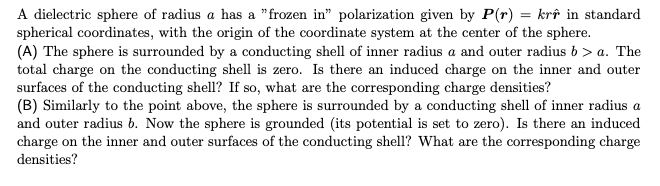A dielectric sphere of radius a has a ”frozen in” polarization given by P (r) = krrˆ in standard spherical coordinates, with the origin of the coordinate system at the center of the sphere. (A) The sphere is surrounded by a conducting shell of inner radius a and outer radius b > a. The total charge on the conducting shell is zero. Is there an induced charge on the inner and outer surfaces of the conducting shell? If so, what...

• ### Problem C. A dipole with dipole moment p pointing towards the positive z direction is placed...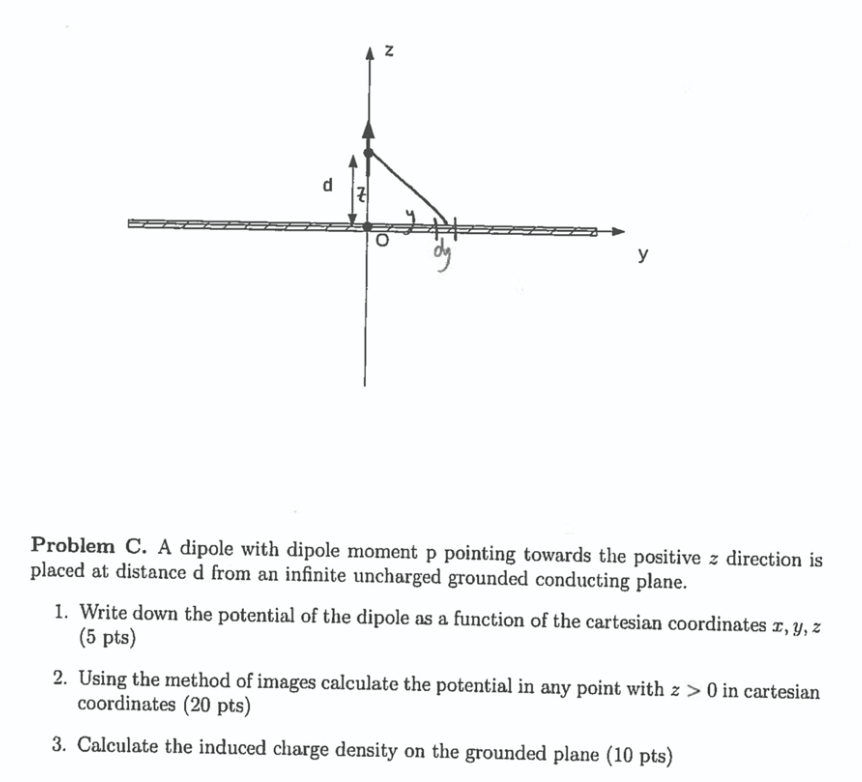Problem C. A dipole with dipole moment p pointing towards the positive z direction is placed at distance d from an infinite uncharged grounded conducting plane. 1. Write down the potential of the dipole as a function of the cartesian coordinates I, y, z (5 pts) 2. Using the method of images calculate the potential in any point with z > 0 in cartesian coordinates (20 pts) 3. Calculate the induced charge density on the grounded plane (10 pts)

Free Homework App